Next: Combined Insertion with Subcycling Up: Dynamic Cohesive Node Insertion Previous: Damping of Blind Insertion   Contents

## Dynamic Insertion with Pre-Stretch

To minimize the nodal oscillations resulting from blind insertion, we employ a pre-stretch to cohesive nodes during insertion. With this pre-stretch the cohesive node is inserted in a state most closely approximating the state it would be in had the insertion occurred at the beginning of the simulation. Using again the reference problem described at the beginning of this chapter, we pre-stretch nodesthroughduring dynamic insertion at the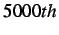and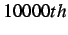time step. The velocity profiles for nodeare presented in in Figures 3.17 and 3.18, for each of the insertions.

Comparing these velocity profiles to the blind insertion profiles in Figures 3.13 and 3.14, we can see that pre-stretching is able to minimize the oscillations quite well. This method is also able to avoid the minor stabilization period that is required with damping. Furthermore, Figures 3.19 and 3.20 plot the separation distance for two cohesive halves of node, for dynamic pre-stretched insertion at thetime step. From these two figures we can clearly see how oscillatory the separations cohesive node are, as well as how significantly pre-stretching minimizes them.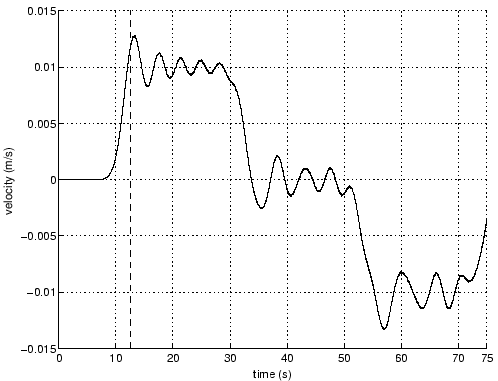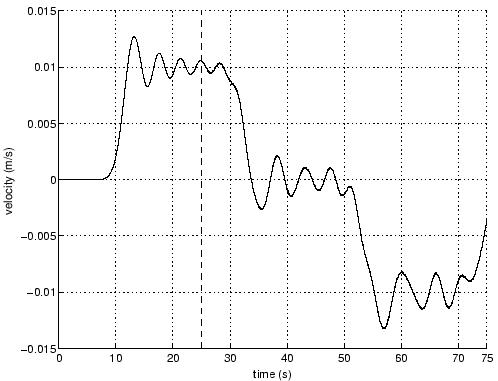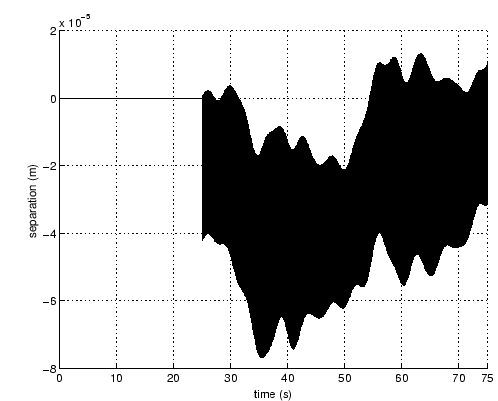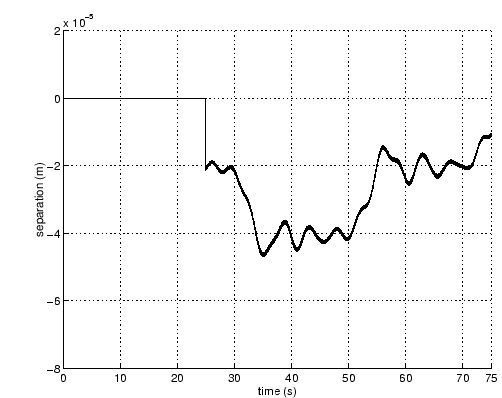In order to obtain timing information we have increased the size of the reference problem to one having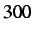segments of length, as seen in Figure 3.21, The middle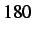nodes are made cohesive at the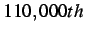time step or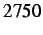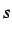for a critical time step reduced by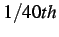to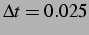The total simulation is run for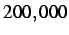time steps ().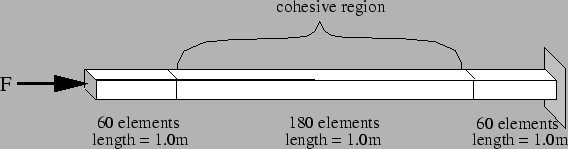Table 3.2 gives the timing results for the cohesive and internal force calculations as well as the total simulation time. We gain a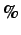time savings in the cohesive calculations which is on par with the approximate time of insertion,time step out of, or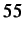through the simulation. The internal force calculations are increased slightly because the number of nodes has increased and hence the number of internal force calculations has also increased.

Table 3.2: Timing results for dynamic cohesive node insertion with pre-stretching. CPU time saving (in %) is given in parentheses.
 Subroutine Reference Case [s] Dynamic Case [s]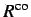9.17 3.82 ( 58%)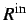6.74 6.88 ( 0%)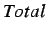60.91 51.34 ( 16%)Next: Combined Insertion with Subcycling Up: Dynamic Cohesive Node Insertion Previous: Damping of Blind Insertion   Contents
Mariusz Zaczek 2002-10-13